Want to share your content on R-bloggers? click here if you have a blog, or here if you don't.

What drives the estimates apart?

## Previously

A post by Investment Performance Guy prompted “Variability of volatility estimates from daily data”.

In my comments to the original post I suggested that using daily data to estimate volatility would be equivalent to using monthly data except with less variability.  Dave, the Investment Performance Guy, proposed the exquisitely reasonable next step: prove it.  (But he phrased it much more politely.)

## Data

Daily closing log returns of the S&P 500 from the start of 1950.

Three-year non-overlapping periods were used.  So the estimates with monthly data use 36 data points, and the daily estimates use about 756 data points.

The monthly estimates are annualized by multiplying the standard deviation by the square root of 12.  The daily estimates are annualized with the square root of 252.

## Differences

Figure 1 shows what I expected to get when comparing the difference between the estimates of volatility (annualized standard deviation in percent) using daily or monthly data.  The line wiggles around zero.

Figure 1: The daily volatility estimate minus the monthly estimate for each three-year period starting in 1950 through 1997.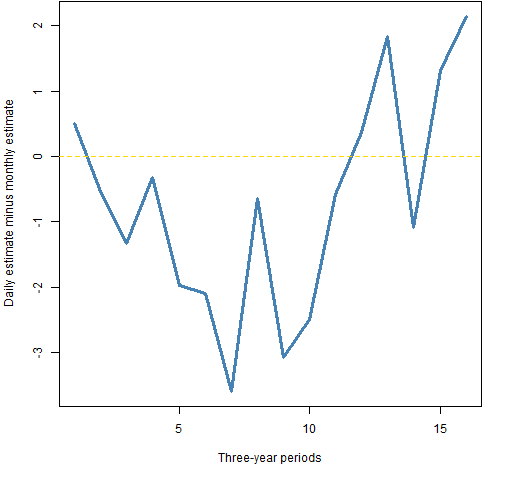Figure 2 shows the full results, and we get a different impression.  In case you didn’t think it before from Figure 1, the run of 10 estimates where daily is less than monthly is fairly extraordinary if they were estimating the same thing.

Figure 2: The daily volatility estimate minus the monthly estimate for each three-year period starting in 1950.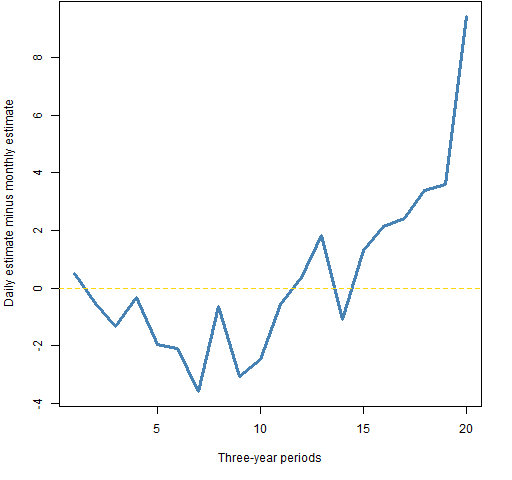Figure 3 moves the windows over by one year.  We get a similar pattern to that in Figure 2.

Figure 3: The daily volatility estimate minus the monthly estimate for each three-year period starting in 1951.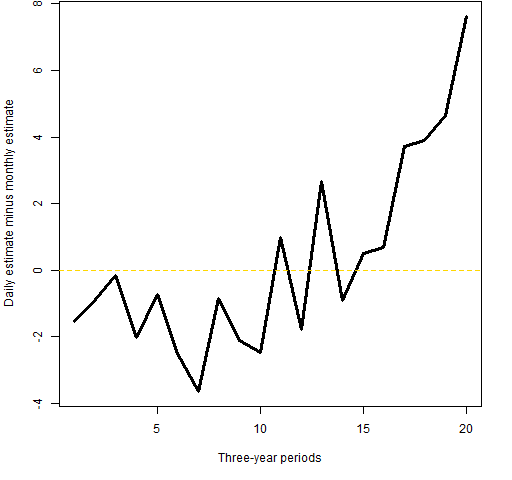The New York Times had a recent piece on “excess volatility” that echoes the results here.  (Note, though, that “excess volatility” is often used in a different sense.)

Figure 4 shows the monthly versus daily estimates for the three-year periods along with 95% bootstrap confidence intervals.

Figure 4: Point estimates and 95% confidence intervals for monthly and daily volatility estimates on three-year periods starting in 1950.The right-most box is for years 2007-2009.  The gigantic box is years 1986-1988 — it is gigantic because there is a data point that is about -23% (daily) or -25% (monthly) which can appear numerous times in a bootstrap sample, or not at all.  The small box that sticks out at the bottom is 2004-2006.

The ratio of the heights of the boxes to their widths shows the advantage of using daily versus monthly data in terms of variability of the estimate.

## Autocorrelation

If the data obeyed the assumptions that statisticians want to have, then the monthly and daily estimates would be giving us the same thing up to estimation error.  The above figures suggest that perhaps they aren’t aiming at the same place — that is, that there’s an assumption that fails.

My original point of view that prompted this post was not that the assumptions held, but that they wouldn’t fail by enough to make a material difference.  I seem to have been wrong.

What we are seeing seems to imply that the S&P random walk is falling off the tightrope — that there is autocorrelation of some sort in the data.

Figure 5 shows the estimate from an AR(1) model on running windows of 250 trading days.  The yellow lines are the 95% confidence interval for randomly sampled daily returns.  The width of true confidence intervals will vary over time, but this gives a rough idea.

Figure 5: autoregression coefficient on running 250-day windows.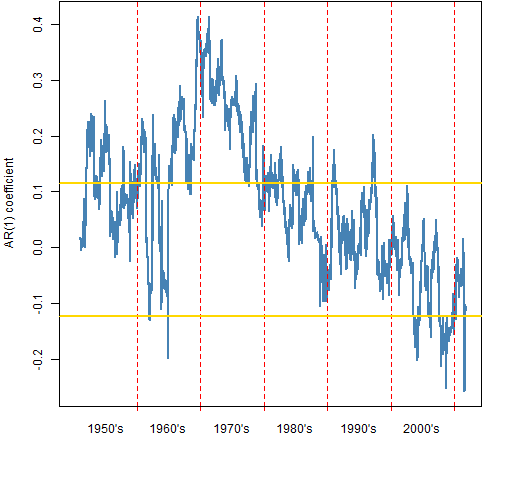Positive autocorrelation implies momentum and that the monthly volatility estimates would tend to be larger than the daily estimates.  Negative autocorrelation implies mean reversion and that the daily estimates would tend to be larger than the monthly estimates.

The positive autocorrelation in decades past might have been due to stale prices, and hence not a money-making opportunity.  However, if that were the case, I would expect it to have been more consistently positive from the start of the data.

The AR(1) model need not be an especially good reflection of the time dependency that is in the returns.  And it probably isn’t.

Figure 6 compares the volatility estimates over time.

Figure 6: Monthly (blue) and daily (black) volatility estimates over each three-year period starting in 1950.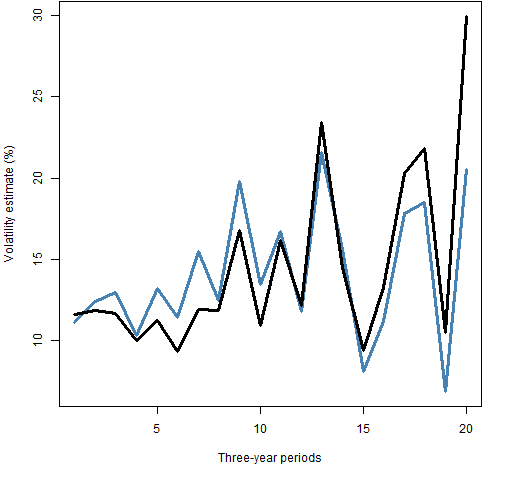## Questions

Is the presumed mean reversion in the market lately a good thing or a bad thing?

Why would it be there?

Is there a way to “properly” annualize volatility?

What is the connection between what we’ve just seen and “Is momentum really momentum?” by Robert Novy-Marx (which comes to us via Whitebox Selected Research)?

## Appendix R

The computations and graphs were (of course) done in R.

#### daily to monthly

The daily returns are just a vector with names in the form of "1950-01-04".  The command to get monthly returns was:

spxmonret <- tapply(spxret, substring(names(spxret),1,7), sum)

This categorizes each observation by month and sums the elements within each month.

#### bespoke functions

The function that does all the work (estimates and confidence intervals) is pp.volcompare.

Figure 4 was created with the aid of function pp.plot2ci and Figure 5 used pp.timeplot.

#### autoregression estimation

The data for Figure 5 was computed with:

spx.ar1 <- spxret
spx.ar1[] <- NA
for(i in 250:length(spxret)) spx.ar1[i] <- ar(spxret[seq(to=i, length=250)], order=1, aic=FALSE)\$ar

spx.ar1boot <- numeric(1e4)
for(i in 1:1e4) spx.ar1boot[i] <- ar(spxret[sample(15548, 250)], order=1, aic=FALSE)\$ar

A simplified version of the command to add the confidence interval to the plot is:

abline(h=quantile(spx.ar1boot, c(.025, .975)))

Subscribe to the Portfolio Probe blog by Email